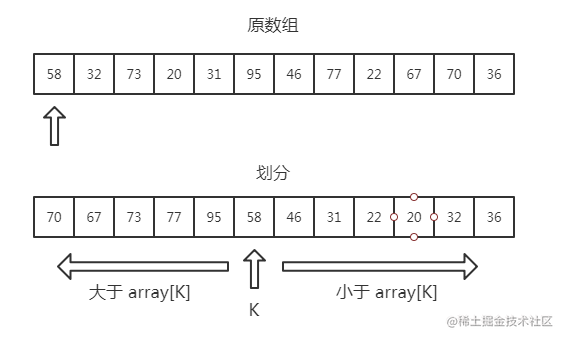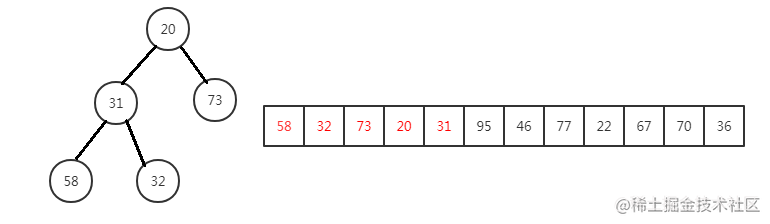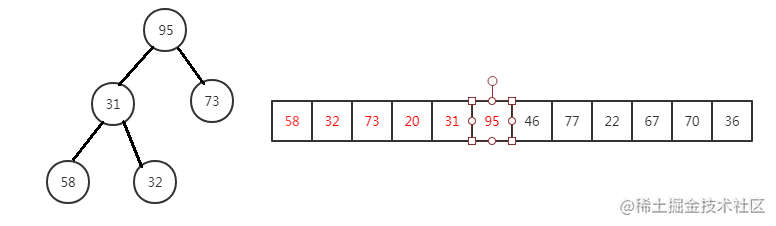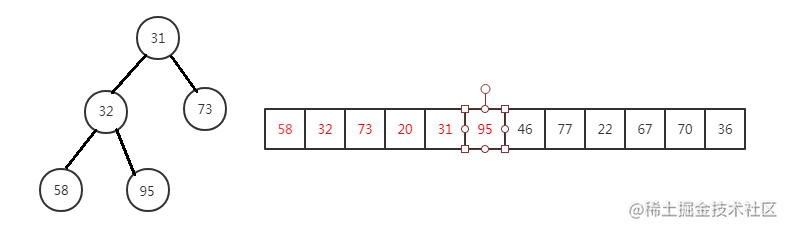# 二. O(n) 时间复杂度的方法# 四. 利用最经典的方法，一台机器也能处理海量数据将数据插入堆95 大于 20，进行替换95 下沉，维持小顶堆

``````public List<Integer> solutionByHeap(int[] input, int k) {
List<Integer> list = new ArrayList<>();
if (k > input.length || k == 0) {
return list;
}
Queue<Integer> queue = new PriorityQueue<>();
for (int num : input) {
if (queue.size() < k) {
} else if (queue.peek() < num){
queue.poll();
}
}
while (k-- > 0) {
}
return list;
}